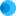Intuit HelpIntuit

# Ending balance sheet is out of balance on Form 1065 in ProConnect Tax

SOLVEDby IntuitProConnect Tax5Updated August 10, 2021Most of the lines on the Schedule L, Balance Sheets per Books are input and output, however, some of the ending amounts are automatically calcuated by ProConnect Tax unless an entry is made in the ending column on the balance sheet input screen.

## To review the balance sheet

1. Go to the Check Return tab.
2. Select Forms > US from the left menu, then select 1065 from the list.
3. Scroll to the Schedule L, Balance Sheets per Books page to determine which line or lines are incorrect or causing the balance sheet to be out of balance.

## To review the input

• Go to the Input Return tab.
• Select Balance Sheet, M-1, M-2 from the left menu.
• Select Balance Sheet, then Federal.
• Make changes as needed to balance the balance sheet.

### Assets

• Inventories - This is based on the Cost of Goods Sold entries.
• Buildings and other depreciable assets - ProConnect Tax will automatically add to the beginning the depreciable assets, any assets placed in service in the current tax year., and will subtract any assets sold during the year to arrive at the end-of-year balance.
• Less accumulated depreciation - the program will automatically enter accumulated depreciation on the balance sheet from assets in the depreciation screen, and subtract any depreciation from assets sold during the year to calculate accumulated depreciation at the end of the year.
• Depletable assets - The ending balance is automatically calculated as follows: beginning balance of depletable assets plus current year change in depletable assets entered in oil and gas.
• Less accumulated depletion - The calculation is based on the entry in Current Year Book Depletion (Table or Dollar Amount). If this field is left blank, federal tax depletion is used to calculate the ending balance of accumulated depletion.
• Intangible assets - The ending balance is automatically calculated as follows: beginning balance of intangible assets plus current year change in intangible assets entered in depreciation.
• For this calculation, an intangible asset entered in depreciation is defined as an asset placed in service during the current year having an entry of 97 in Method, an entry of Amortization in Category, or an entry in the amortization code section.
• Accumulated amortization - The calculation is based on the entry in Current Year Book Amortization (Table or Dollar Amount). If this is left blank, federal tax amortization is used to calculate the ending balance of accumulated amortization.

### Liabilities

• Partners' capital accounts - This amount is automatically calculated on the Schedule M-2. An override is available at the end of the balance sheet section.